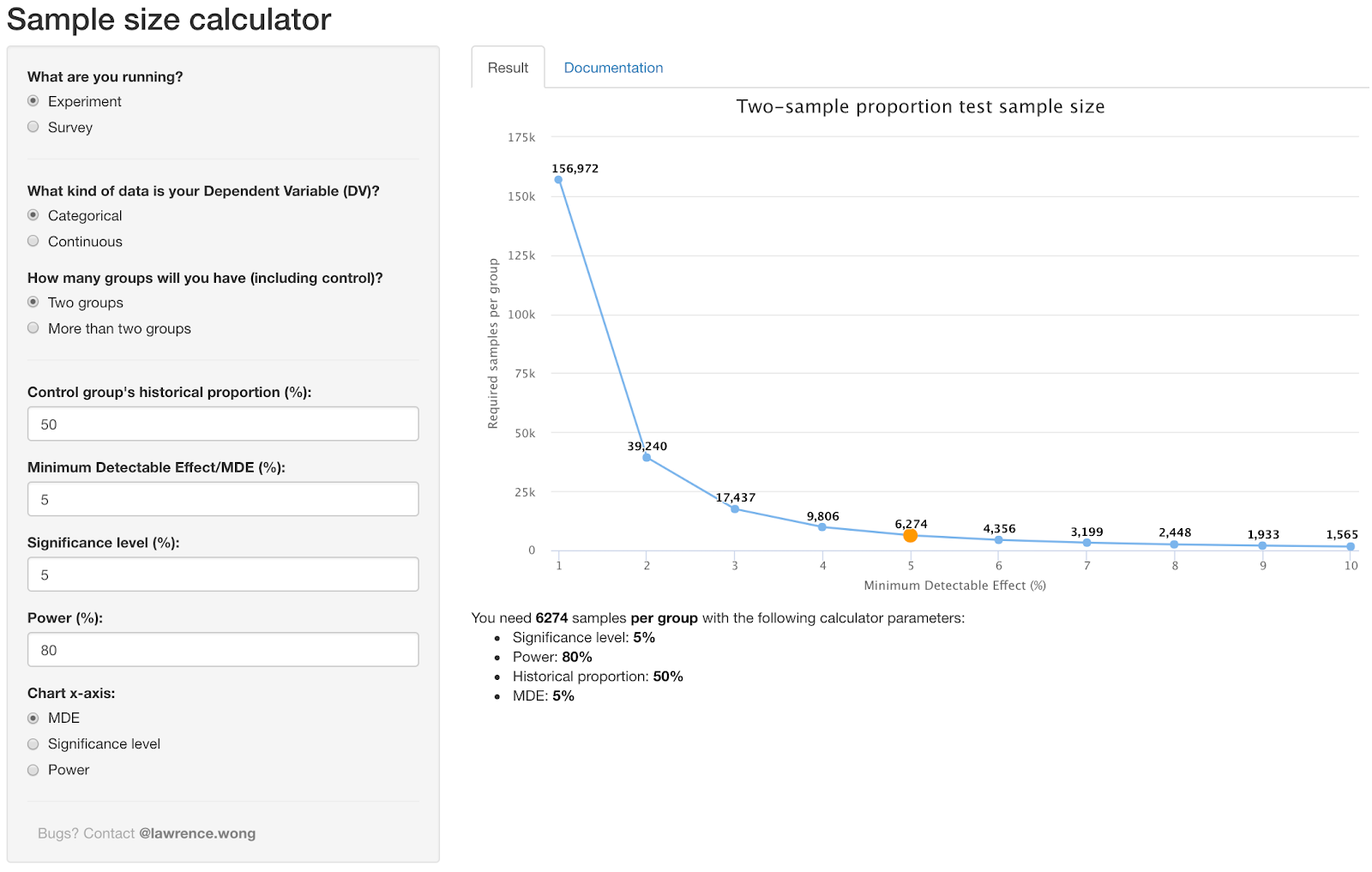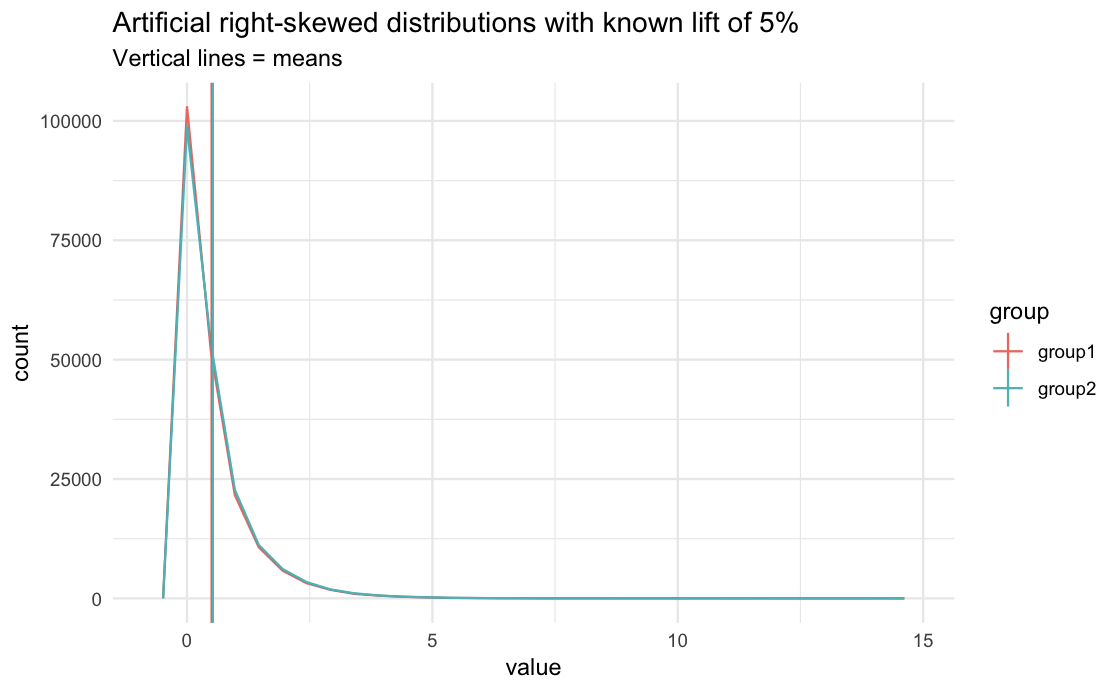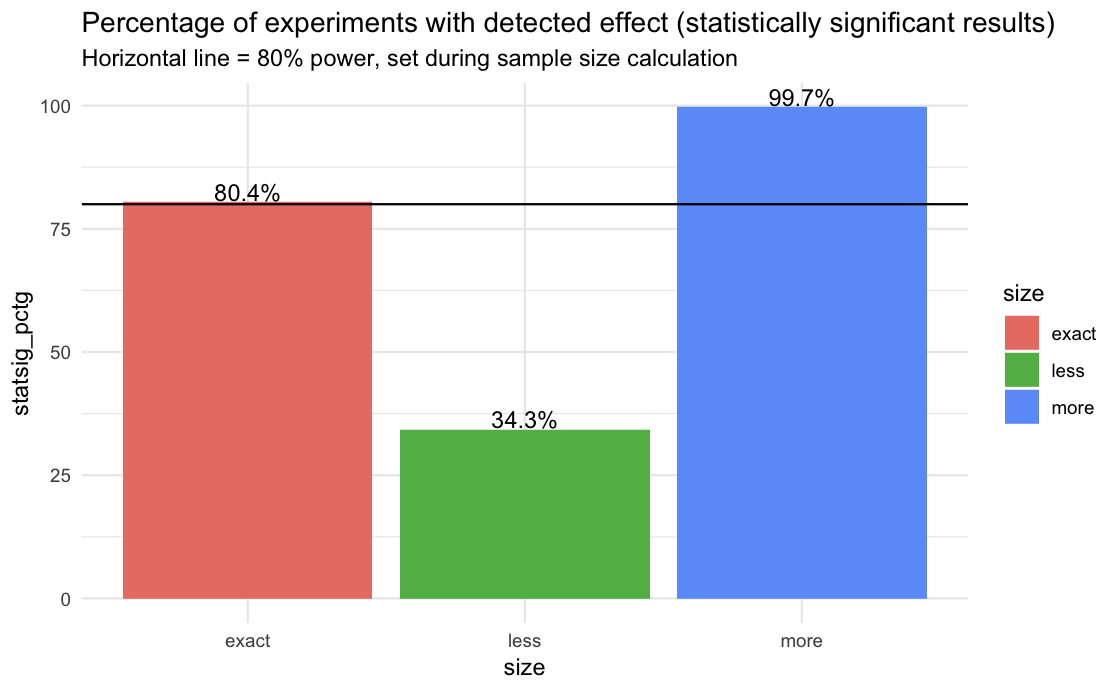# Efficient Experimentation at Gojek

A breakdown of the tool we use to estimate the right audience sample size for our experiments.

By Lawrence Wong

Experiments are wonderful things. They help us validate our hypotheses without having to involve our entire user base. However, any good experiment first requirs the answer to the question — how do we know the right number of people to include in it?

Let’s break down why this is important. If we target too few, our experiments will not detect smaller effects, leading us to miss legitimate opportunities. If we target too many, we would be wasting resources and potentially hurting our business metrics (if the treatments turn out to be detrimental).

Previously, we used simple heuristics (and a mysterious formula) to estimate the required sample size. These practices were not very scientific and produced inconsistent experimental results. To compensate, we had to repeat the same experiment multiple times just to validate the results. Sometimes different iterations would conflict with each other, leaving everyone scratching their heads.

Last year, we ditched those unscientific practices and built a new tool called Sample Size Calculator (yes, imaginative name, we know). This post explains how the calculator helps us find the right number of people to include in experiments.

Our calculator is based on Frequentist school of statistics. Under the hood, we used both the pwr package and the base sample size calculation functions in R programming language. This is what the tool looks like:Figure 1. Screenshot of the Sample Size Calculator used at Gojek

The most important parameters are the type of dependent variable measured, the historical data, and the required sensitivity from the experiment. As you can see in the graph, if we increase sensitivity to allow detection of smaller effects, the required sample size soars, which makes sense.

Although this is a major step in the right direction, there is a problem if we are interested in a continuous dependent variable for our experiment. This is because the underlying methodology used in the calculator assumes a normal distribution, while most continuous metrics that we care about at Gojek — such as `bookings per user` — are usually skewed. By ‘skewed’, we mean that most users are light users and only a small percentage are heavy users. This could cause the computed sample size requirement to be wrong because a key assumption was not met.

We have four options to deal with this problem:

1. Use a methodology that doesn’t assume normality. Unfortunately, the available non-parametric methods are complex to implement  
2. Transform data to have a normal distribution. This doesn’t always work — some data just can’t be coerced to normal
3. Use a larger sample size. Since the worry is lower power than expected, simple multiplication should boost the power, but the multiplier needs to be reasonable. Figuring the right multiplier would be another beast on its own
4. Ignore it. This is the path we took
But but but… that’s not scientific!

Don’t worry. We tested the robustness of this solution before going ahead with it.

As you can see in the graph below, we created two right-skewed distributions with known 5% difference in means to serve as our populations. The plan is to continuously sample from this population (equivalent to replicating the same experiment many times) and see how often we can detect this 5% difference (aka what is our “power”).Figure 2. Two right-skewed distributions (typical shape for continuous metric at Gojek) with known mean difference (lift) of 5%

The first step is to calculate the required sample size with a power of 80% and minimum detectable effect of 5%. Let’s refer to this sample size requirement as the “exact” size. Then we divide and multiply it by three and call them “less” and “more”, respectively. For each sample size, we sample from the populations, compute the mean difference (typically called “lift”), and repeat this procedure many times.

The resulting distributions of lifts are shown below. The coloured vertical lines show the average lift of each experimental size, which is also equal to the actual population lift. This is central limit theorem at work. What is interesting are the relative shapes of the sampling distributions. The “more” group is the safest and most consistent but the “exact” group seems to have good accuracy with only a third of the sample size. In the “less” group, we even see a non-negligible number of lifts that are negative, even though we know the population lift is actually +5%. This is in line with our intuition that larger sample size is generally more trustworthy than a smaller one, all else being equal.

Since we currently report the statistical significance of our experimental results at Gojek, we will also run t-test for each of our sample here.

As it turns out, 80.4% of the experiments in the “exact” group have statistically significant results (p < 0.05), which is almost equal to the 80% power we set in the sample size calculator. In layman’s terms, the tool roughly promised that if you run the experiment with this sample size, you have 80% chance of detecting a 5% lift (the minimum detectable effect) if it’s there.

Since it kept its promise even on non-normally distributed data, we can conclude that the methodology is robust to some degree of violation of the normality assumption. Therefore we can ignore it until otherwise proven.Figure 4. Percentage of samples/experiments with statistically significant results (p < 0.05) from each size group

Meanwhile, the “less” group had only ~34% statistically significant results, suggesting that we shouldn’t run an experiment if we cannot fulfil the sample size requirement — since we risk not being able to detect an existing effect. With the “more” group having close to 100% statistically significant results, we again reaffirm that larger sample size is generally a good thing if we can afford it.

Since it was deployed in mid-2018, many teams across Gojek have adopted the Sample Size Calculator. So far, nearly 1,000 experiments have incorporated this tool in their designs, and it is also a part of Litmus, our experimentation platform. The more critical takeaway for us is that the experiments run using the recommended sample sizes have similar results when full-scaled. This fact has enabled us to iterate faster and cut waste while maintaining a high degree of confidence in the experimental results. ✌️

Did we mention Gojek’s Growth team is hiring analysts? We are a super data-driven team and have helped shape some of the company’s best practices through projects like this. If you are analytical, interested in sharpening your technical chops, and want to have a meaningful impact to Gojek’s hypergrowth, come join us!